#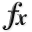N-D Test Functions U¶

class go_benchmark.Ursem01(dimensions=2)

Ursem 1 test objective function.

This class defines the Ursem 1 global optimization problem. This is a unimodal minimization problem defined as follows:Here,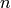represents the number of dimensions and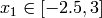,.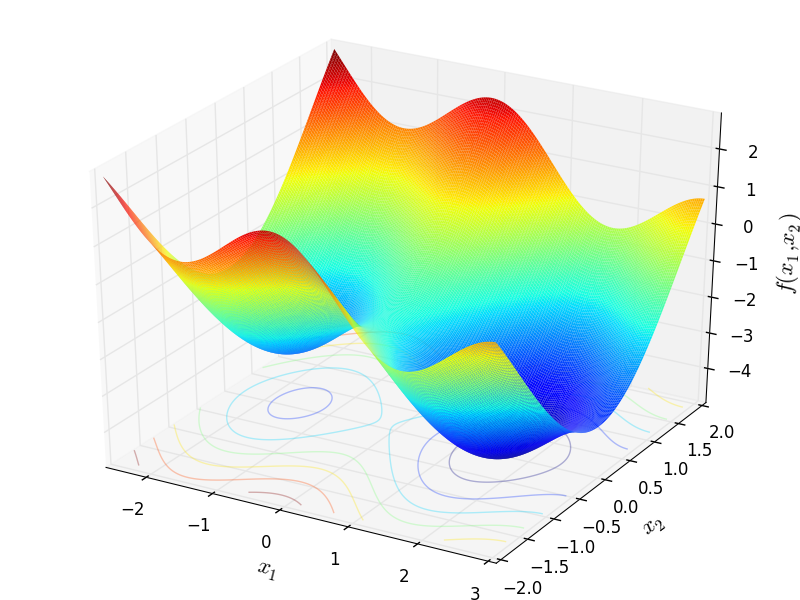Two-dimensional Ursem 1 function

Global optimum:forclass go_benchmark.Ursem03(dimensions=2)

Ursem 3 test objective function.

This class defines the Ursem 3 global optimization problem. This is a multimodal minimization problem defined as follows: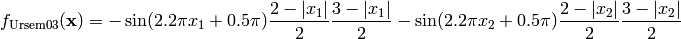Here,represents the number of dimensions and,.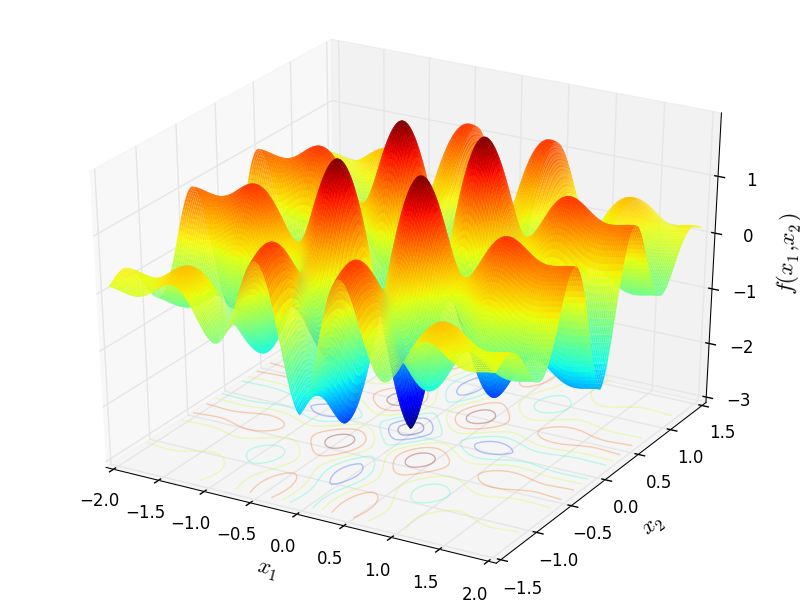Two-dimensional Ursem 3 function

Global optimum: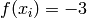for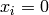for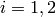class go_benchmark.Ursem04(dimensions=2)

Ursem 4 test objective function.

This class defines the Ursem 4 global optimization problem. This is a multimodal minimization problem defined as follows:Here,represents the number of dimensions and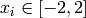for.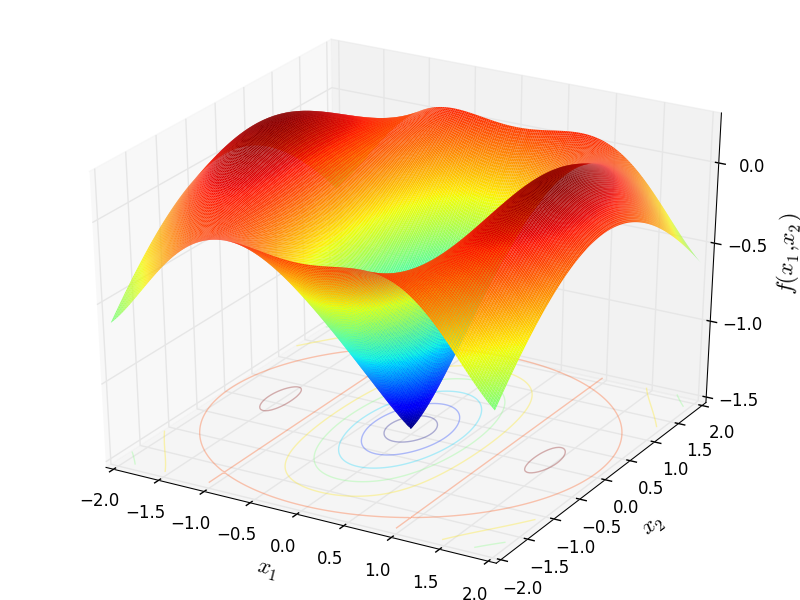Two-dimensional Ursem 4 function

Global optimum: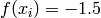forforclass go_benchmark.UrsemWaves(dimensions=2)

Ursem Waves test objective function.

This class defines the Ursem Waves global optimization problem. This is a multimodal minimization problem defined as follows: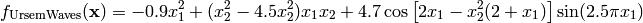Here,represents the number of dimensions and,.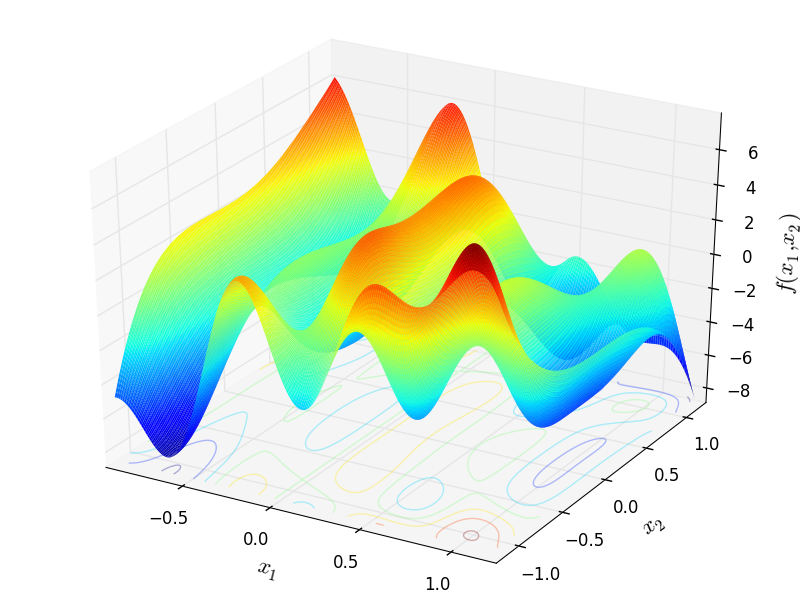Two-dimensional Ursem Waves function

Global optimum: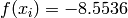for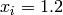for#### Previous topic

N-D Test Functions T

#### Next topic

N-D Test Functions V